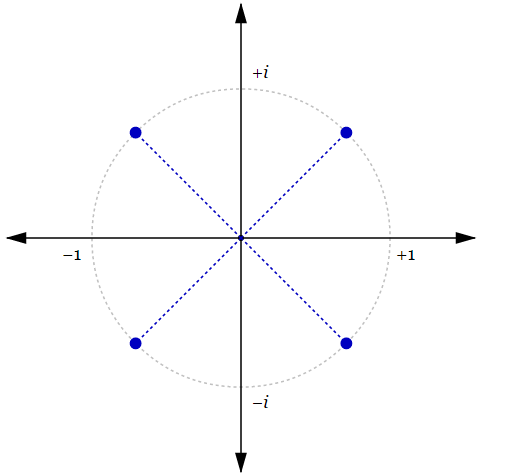Algebra Level 5$\large(x+3)^4+(x+5)^4=20$

Find the sum of all real roots of the equation above.